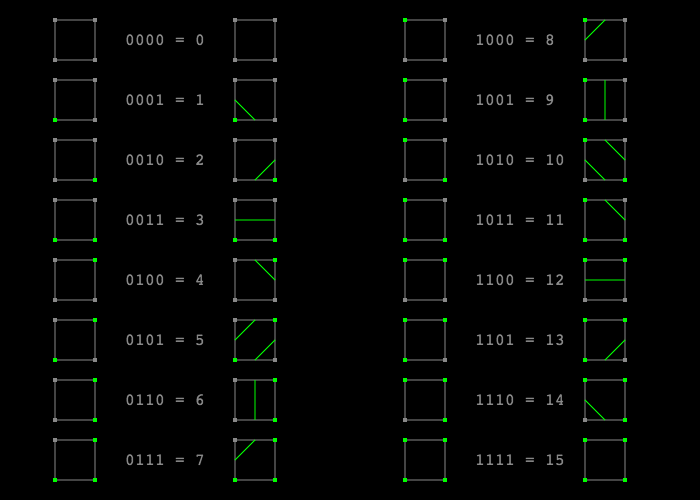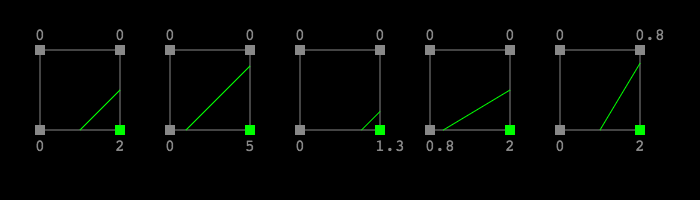# Metaballs and Marching Squares

Something about making visually interesting simulations to play with just gets me really excited about programming, particularly when there’s some cool algorithm or bit of math backing it.

Reading a bit about particle simulations on Wikipedia, I stumbled upon metaballs. In 3D, metaballs looks something like this:

This looked like it had the potential to yield some cool behaviour, so I decided to dive in. To start, I just wanted some circles bouncing around, like this:

Sweet. The next step is to make these things blobby.

# The Math

As a bit of a refresher, this is the equation of a circle centered at $$(x_0, y_0)$$ with radius $$r$$:

$$(x - x_0)^2 + (y - y_0)^2 = r^2$$

Or if you wanted to model all the points inside or on the boundary the circle, it would be this:

$$(x - x_0)^2 + (y - y_0)^2 \leq r^2$$

Rearranging this a little, we get this:

$$\frac{r^2}{(x - x_0)^2 + (y - y_0)^2} \geq 1$$

When we have a bunch of circles bouncing around, we can model all the points inside of any of the circles like this, if circle $$i$$ is defined by radius $$r_i$$, and center $$(x_i, y_i)$$:

$$\max_{i=0}^n \frac{r_i^2}{(x - x_i)^2 + (y - y_i)^2} \geq 1$$

The thing that makes metaballs all blobby-like is that instead of considering each circle separately, we take contributions from each circle. This models all of the points inside of all the metaballs:

$$\sum_{i=0}^n \frac{r_i^2}{(x - x_i)^2 + (y - y_i)^2} \geq 1$$

We’re going to be using the left hand side of this inequality quite a bit, so let’s say:

$$f(x, y) = \sum_{i=0}^n \frac{r_i^2}{(x - x_i)^2 + (y - y_i)^2}$$

If we sample the $$f(x,y)$$ in a grid and plot the results, we get something like this:

If we increase the resolution, we start getting a kind of amoeba-blobby effect.

You might be thinking that this still looks a little blocky. We sampled in a grid, so why didn’t we just sample every pixel instead to make it look smoother?

Well, for 40 bouncing circles, on a 700x500 grid, that would be on the order of 14 million operations. If we want to have a nice smooth 60fps animation, that would be 840 million operations per second. JavaScript engines may be fast nowadays, but not that fast.

Thankfully, there’s a much nicer algorithm to get a smooth approximation of the boundary dividing the area where the inequality holds from the area where it doesn’t.

# Marching Squares

The Marching Squares algorithm generates an approximation for a contour line of a two dimensional scalar field. Put in another way, if we have a 2D function, this will find an approximation of a line where all points on the line have the same function value. In our case, we’re trying to find the outlines where

$$f(x, y) = 1$$

Instead of each cell being drawn based on one sample in that cell, we’re going to sample the corners of each cell, then do something more intelligent to draw a line in some cells, instead of just filling in the entire square.

Sampling the corners looks like this:

The “do something more intelligent” relies on the fact that in every case other than all corners having $$f(x, y) \geq 1$$ or all corners having $$f(x, y) \lt 1$$ (cases 0 and 15 in the diagram below), we know that part of the boundary of our blobs will run through that cell.

Since there are only 2^4 possible configurations of a cell’s corners, we can just enumerate them all.The configuration number between 0-15 is computed by assigning a value of 0 to each of the corners where $$f(x, y) \lt 1$$, and a value of 1 where $$f(x, y) \geq 1$$, then interpreting these bits as a binary number, ordered (southwest, southeast, northeast, northwest).

To the keen observer, you’ll notice that configurations 5 and 10 are ambiguous, because we can’t be sure if the center of the cell is inside our boundary or outside it. For the sake of simplicity we just assume it’s always inside the boundary.

When we perform this mapping process for all of the cells in the grid, we end up with something like this:

If we increase the resolution and get rid of our debugging information, we wind up with this:

This looks a little nicer than the solid blocks we had before, but all we’ve really done is turned some of our 90 degree angles into 45 degree angles. We should be able to get smoother without increasing the sampling resolution.

# Linear Interpolation

When we categorized our cells into one of 16 types, we only retained one bit of information from each corner. We can use the original sample values to give us a much better approximation for where the contour line really intersects the cell.

Consider these examples, and see how even though these are all of the same 0-16 type, the underlying samples should intuitively result in noticeably different lines.Even though each of these cells would be classified as type "0100 = 2", the green line approximating the set of points within the cell where $$f(x, y) = 1$$ can vary dramatically depending on the underlying sample values.

To calculate how to adjust these lines based on the original corner sample values, we’re going to apply linear interpolation. Given the point labels in this diagram:Let’s work through the math for finding $$(Q_x, Q_y)$$. The process would be the same for $$(P_x, P_y)$$ or the point on either of the other 2 sides in the case of other 0-15 cell configurations.

For starters, we know this just looking at the diagram:

$$Q_x = B_x$$

And, even though the $$f(x, y)$$ isn’t linear, linear interpolation still gives a good enough result to provide us with visually meaningful smooth contour lines, so we can say:

$$\frac{Q_y - B_y}{D_y - B_y} \approx \frac{f(Q_x, Q_y) - f(B_x, B_y)} {f(D_x, D_y) - f(B_x, B_y)}$$

Since we’re trying to get $$Q$$ to lie roughly on the counter line where $$f(x, y) = 1$$, we want $$f(Q_x, Q_y) \approx 1$$, so let’s substitute that and rearrange.

$$Q_y = B_y + (D_y - B_y) \left( \frac{1 - f(B_x, B_y)}{f(D_x, D_y) - f(B_x, B_y)} \right)$$

All the values on the right hand side are known, so we can just plug them in. The result is something like this:

And if we crank up the resolution and hide the debug info, we’re left with this pretty result:

As a bonus, I also implemented the blocky version of this (no marching squares) on the Khan Academy Computer Science platform, using Voronoi diagram-esque coloring for the cells.

# Applications

It turns out that this algorithm can be actually useful!

The most likely example of this you’ve seen are elevation contours on maps. Before we had crazy high resolution satellite imaging of everything, we could’ve taken elevation samples in a grid, then drawn contour lines for a fixed number of elevations to create maps like this:

Another application of this you might’ve seen without realizing it is applying tilesets in games. There’s a great explanation of that in a blog post called Squares Made for Marching on Project Renegade. Linear interpolation doesn’t apply to this version of the algorithm.